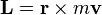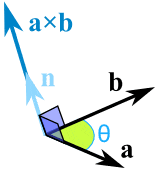# Law of conservation of Angular Momentum

• avito009
This is true if the velocity remains constant. If the velocity changes, then the angle between the vectors changes, and the law of conservation of angular momentum would not apply.f

#### avito009

Keplers second law: An imaginary line joining a planet and the sun sweeps out an equal area of space in equal amounts of time.

So this shows that the Earth would move faster if it was near the sun. Why?

I have read that an abject will move faster if the mass has become smaller. If mass has increased it will move slower thus angular momentum would be conserved.

So in this case the Earth is near the sun. So its weight should increase. If weight increases it should move slowly. So how does the Earth moving faster conserve angular momentum?

Keplers second law: An imaginary line joining a planet and the sun sweeps out an equal area of space in equal amounts of time.

So this shows that the Earth would move faster if it was near the sun. Why?
To sweep out an equal area in the same time, a shorter line must move faster.

I have read that an abject will move faster if the mass has become smaller. If mass has increased it will move slower thus angular momentum would be conserved.
Where did you read this? In any case, this is irrelevant as the mass of the Earth doesn't change as it moves closer.

So in this case the Earth is near the sun. So its weight should increase. If weight increases it should move slowly.
The gravitational force acting on the Earth from the sun (the Earth's "weight", if you like) does increase as the Earth nears the sun. But that has nothing to do with its mass, which remains constant.

So how does the Earth moving faster conserve angular momentum?
Do you understand the definition of angular momentum?

Does that mean that no matter how much speed Earth has when it is near or away from the sun. It will take 365 days for it to make one orbit round the sun. Does this explain that the momentum remains the same since equal area is swept by the Earth?

Yeah, that's not how it works at all... A year would be far smaller number of "days" compared to our normal year. The revolutions would change so a "day" would be shorter and a year would be much shorter. The conservation is that the balance stays the same.

Keplers second law: An imaginary line joining a planet and the sun sweeps out an equal area of space in equal amounts of time.

So this shows that the Earth would move faster if it was near the sun. Why?
Kepler's second law does NOT say that the Earth would move faster if its orbit were moved closer to the sun. It says that the Earth moves faster along the orbital path that it already has at places where its trajectory is closer to the sun and slower at places where its trajectory is farther from the sun.

If you were to move the Earth from its current near-circular orbit to a new near-circular orbit closer to the sun then you would need to reduce its angular momentum to do so. It would be sweeping out a lesser area in an equal amount of time.

If you were to move the Earth from its current near-circular orbit to a new near-circular orbit farther from the sun then you would need to increase its angular momentum to do so. It would be sweeping out a greater area in an equal amount of time.

In its existing near-circular orbit, the Earth has a constant angular momentum. The central force from the sun applies no torque. So it sweeps out equal areas in equal times.

•Doc Al
I have understood Law of conservation of angular momentum. If the distance r increases in the below equation then v decreases. r is the distance from the point of rotation and v is the velocity, also m is the mass.If a skater pulls out her arms and spins then r would be 4 and v would be 2. Then when the skater pulls in her arms and spins then r would be 2 and v would be 4. So angular momentum is conserved it always equals 8 in this example assuming mass is 1 kg.

There does not seem to be a question here. However, two things. First, this:
If the distance r increases in the below equation then v decreases
is not necessarily true. If r increases and m is fixed, it is not necessary that the magnitude of v must decrease if the angle between r and v changes suitably. For instance, you can verify with basic trigonometry that L is constant is for a free particle traveling in a straight line at constant velocity (i.e. subject to no forces), as must be the case. In this case, the magnitude of v does not change but the angle between the two vectors changes such the cross product stays constant. If the angle is held fixed, such as for a particle orbiting in a circle, then indeed the magnitude of v must decrease when r increases.
Second, that formula for angular momentum applies to point masses, not extended bodies like skaters. The basic lesson you are drawing is correct, but to show it rigorously you need the more general formula for angular momentum in terms of moments of inertia.

I have read that an abject will move faster if the mass has become smaller. If mass has increased it will move slower thus angular momentum would be conserved.

Yeah, that's not how it works at all... A year would be far smaller number of "days" compared to our normal year. The revolutions would change so a "day" would be shorter and a year would be much shorter. The conservation is that the balance stays the same.
The time it takes the Earth to rotate on its axis is unrelated to the distance from the sun, so doesn't change here.

LastOneStanding Can you explain how the cross product stays the same even if the angles between the vectors v and r change? Let's take an example.

We can calculate the Cross Product this way:a × b = |a| |b| sin(θ) n

now let's substitute the values

2*3*sin30n= 6*1n=6n

Now when the angle between r and v changes let's say now it is 60. So now

2*3*sin90n= 2*3*-0.3n= -1.8n

So how does the cross product stay same?

Now when the angle between r and v changes let's say now it is 60. So now

2*3*sin90n= 2*3*-0.3n= -1.8n
sin 90 = -0.3?!

So how does the cross product stay same?
If you change the direction of the velocity by applying a torque, you will have changed the angular momentum. The cross product will not, in general, stay the same.

If you change the direction of the velocity without applying a torque, then the change in velocity and the change in angle will work out right so that angular momentum will be conserved and the cross product will remain the same.

a × b = |a| |b| sin(θ) n

now let's substitute the values

2*3*sin30n= 6*1n=6n

Now when the angle between r and v changes let's say now it is 60. So now

2*3*sin90n= 2*3*-0.3n= -1.8n

So how does the cross product stay same?

I don't think your math is correct... sin30 is not 1, and sin60 is not -0.3.

LastOneStanding Can you explain how the cross product stays the same even if the angles between the vectors v and r change? Let's take an example.

We can calculate the Cross Product this way:a × b = |a| |b| sin(θ) n

now let's substitute the values

2*3*sin30n= 6*1n=6n

Now when the angle between r and v changes let's say now it is 60. So now

2*3*sin90n= 2*3*-0.3n= -1.8n

So how does the cross product stay same?
Putting your math errors aside, the cross product ##\vec{r} \times m\vec{v}## stays the same when angular momentum is conserved. That does not mean you can take two vectors, change the angle between them, and expect their cross product to remain the same. Of course not!

The point being made was that not only can ##r## and ##v## change, but the angle between them can change as well. When angular momentum is conserved, if the angle between the vectors changes, then one or both of the magnitudes must also change to compensate.